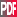18th Conference on Probability and Statistics in the Atmospheric Sciences

9.3

Error propagation through principal components

PAPER WITHDRAWN

G. Louis Smith, National Institute of Aerospace, Hampton, VA

Principal component (PC) analysis is a valuable tool in climatology studies for investigating patterns of variations in time and space. With a series of maps of a parameter, one can compute the PCs which describe the time variations and empirical orthogonal functions (EOFs) which describe the corresponding spatial variations. The question arises as to how measurement errors propagate through these computations to affect the final PC and EOF results. This paper presents a linearized analysis for these errors and demonstrates the resulting equations by applying them to an example, whereby a principal component analysis is applied to the annual cycles of the geographic distribution of longwave surface radiation.

The measurement errors are modeled as consisting of a bias plus uncorrelated errors. The bias errors appear in the mean distribution and do not appear in the PCs or EOFs. The variances of the error in an eigenvalue is proportional to the variance of the measurement errors times the eigenvalue and inversely proportional to the number of regions. Errors in the PCs and EOFs are written in terms of contamination of PCs by each other due to the measurement errors, as quantified by interaction coefficients. These coefficients are proportional to the sum of the eigenvalues of the contaminating and contaminated PC and inversely proportional to the square of the difference of the eigenvalues. The variances of the errors of the PCs are expressed in terms of the PCs, and the variances of errors in the PCs are proportional to the variance of the measurement errors and the interaction coefficients and inversely proportional to the number of regions. The variances of the errors in the EOFs, describing the spatial patterns, are due to the measurement errors directly and also to the contamination of EOFs by each other due to the measurement errors. This contamination in the spatial domain is also described by the interaction coefficients. The interaction coefficients increase quickly with increasing order of the contaminated PC or EOF, so that the growth of errors in PCs and EOFs with order is very rapid and the high order PCs and EOFs become overwhelmed by these errors.

In the example, it is seen that the errors in the first PC are largest (smallest) where the magnitude of the second PC is largest (smallest).Extended Abstract (44K)

Session 9, Statistical Climatology
Thursday, 2 February 2006, 8:45 AM-12:30 PM, A304

Previous paper  Next paper

Browse or search entire meeting

AMS Home Page